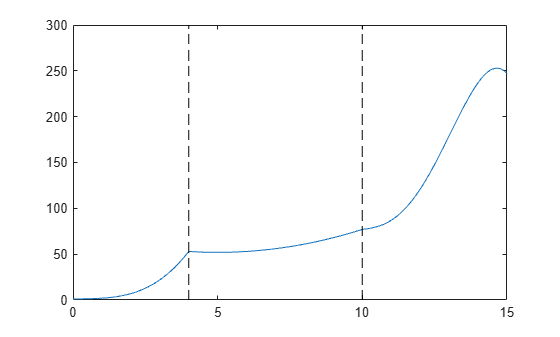mkpp

Make piecewise polynomial

Description

example

pp = mkpp(breaks,coefs) builds a piecewise polynomial pp from its breaks and coefficients. Use ppval to evaluate the piecewise polynomial at specific points, or unmkpp to extract details about the piecewise polynomial.

pp = mkpp(breaks,coefs,d) specifies that the piecewise polynomial is vector-valued, such that the value of each of its coefficients is a vector of length d.

Examples

collapse all

Create a piecewise polynomial that has a cubic polynomial in the interval [0,4], a quadratic polynomial in the interval [4,10], and a quartic polynomial in the interval [10,15].

breaks = [0 4 10 15];
coefs = [0 1 -1 1 1; 0 0 1 -2 53; -1 6 1 4 77];
pp = mkpp(breaks,coefs)
pp = struct with fields:
form: 'pp'
breaks: [0 4 10 15]
coefs: [3x5 double]
pieces: 3
order: 5
dim: 1

Evaluate the piecewise polynomial at many points in the interval [0,15] and plot the results. Plot vertical dashed lines at the break points where the polynomials meet.

xq = 0:0.01:15;
plot(xq,ppval(pp,xq))
line([4 4],ylim,'LineStyle','--','Color','k')
line([10 10],ylim,'LineStyle','--','Color','k')Create and plot a piecewise polynomial with four intervals that alternate between two quadratic polynomials.

The first two subplots show a quadratic polynomial and its negation shifted to the intervals [-8,-4] and [-4,0]. The polynomial is

$1-{\left(\frac{x}{2}-1\right)}^{2}=\frac{-{x}^{2}}{4}+x.$

The third subplot shows a piecewise polynomial constructed by alternating these two quadratic pieces over four intervals. Vertical lines are added to show the points where the polynomials meet.

subplot(2,2,1)
cc = [-1/4 1 0];
pp1 = mkpp([-8 -4],cc);
xx1 = -8:0.1:-4;
plot(xx1,ppval(pp1,xx1),'k-')

subplot(2,2,2)
pp2 = mkpp([-4 0],-cc);
xx2 = -4:0.1:0;
plot(xx2,ppval(pp2,xx2),'k-')

subplot(2,1,2)
pp = mkpp([-8 -4 0 4 8],[cc;-cc;cc;-cc]);
xx = -8:0.1:8;
plot(xx,ppval(pp,xx),'k-')
hold on
line([-4 -4],ylim,'LineStyle','--')
line([0 0],ylim,'LineStyle','--')
line([4 4],ylim,'LineStyle','--')
hold offInput Arguments

collapse all

Break points, specified as a vector of length L+1 with strictly increasing elements that represent the start and end of each of L intervals.

Data Types: single | double

Polynomial coefficients, specified as an L-by-k matrix with the ith row coefs(i,:) containing the local coefficients of an order k polynomial on the ith interval, [breaks(i), breaks(i+1)]. In other words, the polynomial is coefs(i,1)*(X-breaks(i))^(k-1) + coefs(i,2)*(X-breaks(i))^(k-2) + ... + coefs(i,k-1)*(X-breaks(i)) + coefs(i,k).

Data Types: single | double

Dimension, specified as a scalar or vector of integers. Specify d to signify that the piecewise polynomial has coefficient values of size d.

Data Types: single | double

Output Arguments

collapse all

Piecewise polynomial, returned as a structure. Use this structure with the ppval function to evaluate the piecewise polynomial at one or more query points. The structure has these fields.

FieldDescription
form

'pp' for piecewise polynomial

breaks

Vector of length L+1 with strictly increasing elements that represent the start and end of each of L intervals

coefs

L-by-k matrix with each row coefs(i,:) containing the local coefficients of an order k polynomial on the ith interval, [breaks(i),breaks(i+1)]

pieces

Number of pieces, L

order

Order of the polynomials

dim

Dimensionality of target

Since the polynomial coefficients in coefs are local coefficients for each interval, you must subtract the lower endpoint of the corresponding knot interval to use the coefficients in a conventional polynomial equation. In other words, for the coefficients [a,b,c,d] on the interval [x1,x2], the corresponding polynomial is

$f\left(x\right)=a{\left(x-{x}_{1}\right)}^{3}+b{\left(x-{x}_{1}\right)}^{2}+c\left(x-{x}_{1}\right)+d\text{\hspace{0.17em}}.$